# 3 DIFFERENTIATION RULES DIFFERENTIATION RULES 3 2 The

• Slides: 41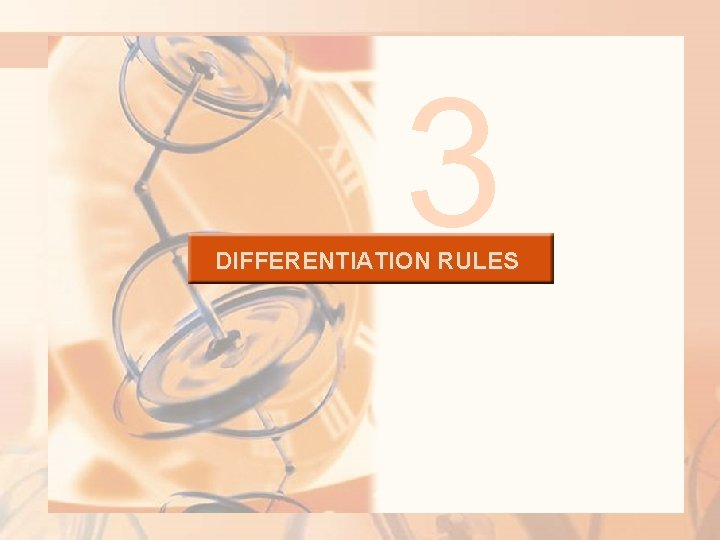3 DIFFERENTIATION RULES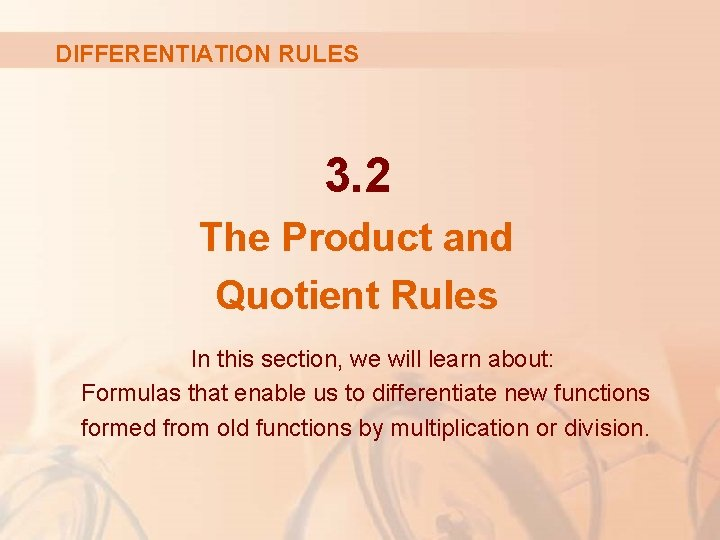DIFFERENTIATION RULES 3. 2 The Product and Quotient Rules In this section, we will learn about: Formulas that enable us to differentiate new functions formed from old functions by multiplication or division.THE PRODUCT RULE By analogy with the Sum and Difference Rules, one might be tempted to guess—as Leibniz did three centuries ago—that the derivative of a product is the product of the derivatives. § However, we can see that this guess is wrong by looking at a particular example.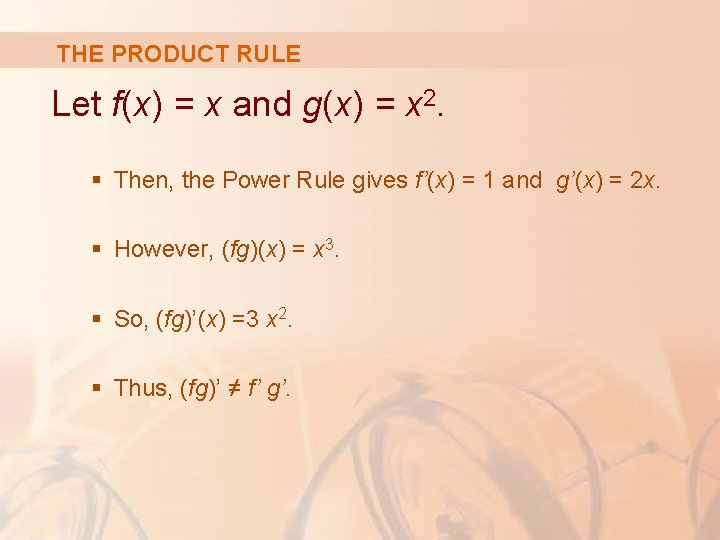THE PRODUCT RULE Let f(x) = x and g(x) = x 2. § Then, the Power Rule gives f’(x) = 1 and g’(x) = 2 x. § However, (fg)(x) = x 3. § So, (fg)’(x) =3 x 2. § Thus, (fg)’ ≠ f’ g’.THE PRODUCT RULE The correct formula was discovered by Leibniz (soon after his false start) and is called the Product Rule.THE PRODUCT RULE Before stating the Product Rule, let’s see how we might discover it. We start by assuming that u = f(x) and v = g(x) are both positive differentiable functions.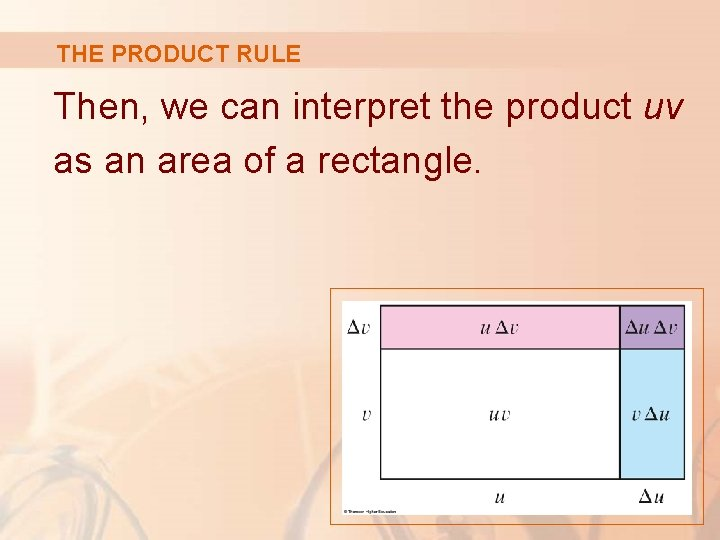THE PRODUCT RULE Then, we can interpret the product uv as an area of a rectangle.THE PRODUCT RULE If x changes by an amount Δx, then the corresponding changes in u and v are: § Δu = f(x + Δx) - f(x) § Δv = g(x + Δx) - g(x)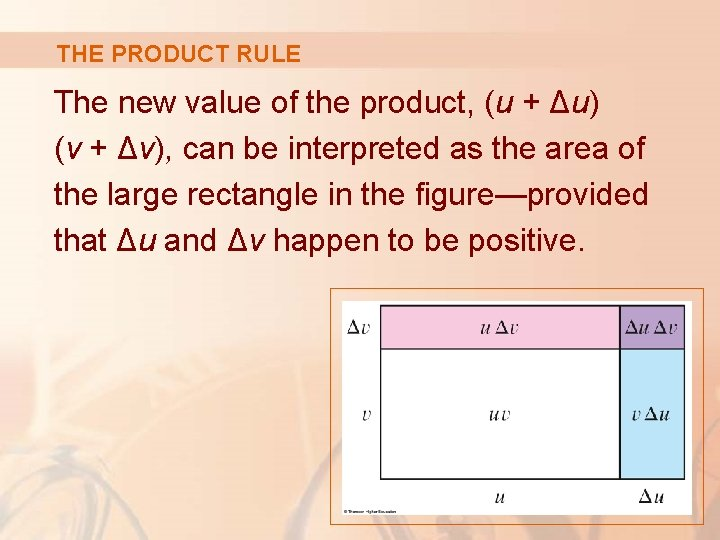THE PRODUCT RULE The new value of the product, (u + Δu) (v + Δv), can be interpreted as the area of the large rectangle in the figure—provided that Δu and Δv happen to be positive.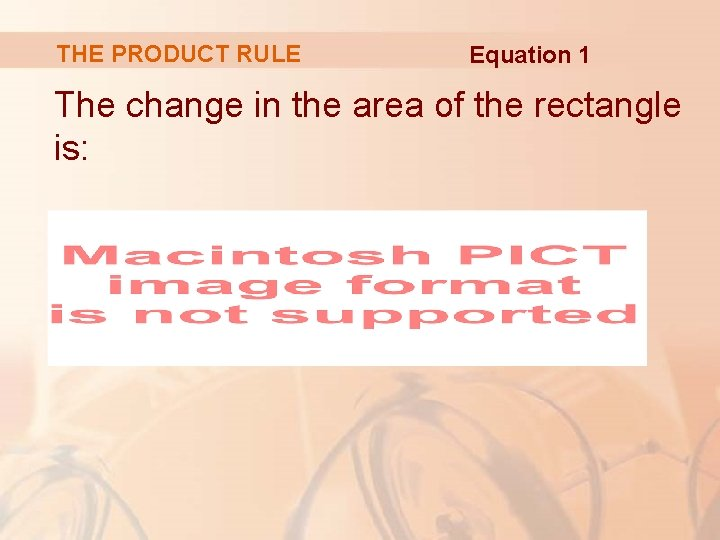THE PRODUCT RULE Equation 1 The change in the area of the rectangle is: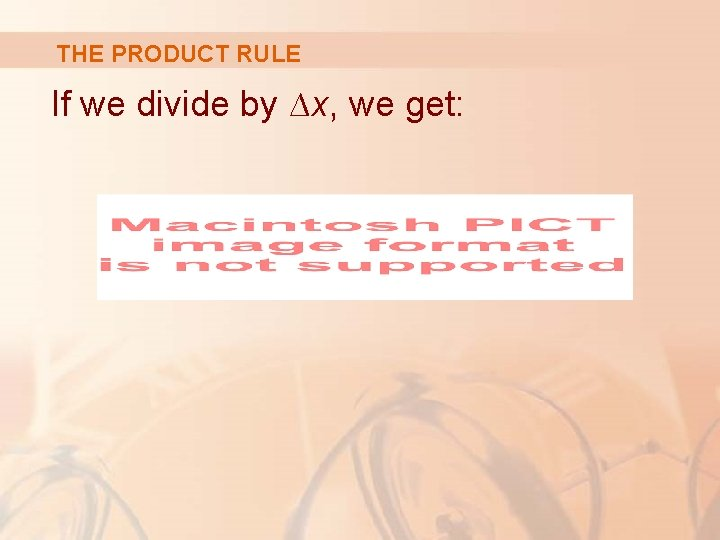THE PRODUCT RULE If we divide by ∆x, we get: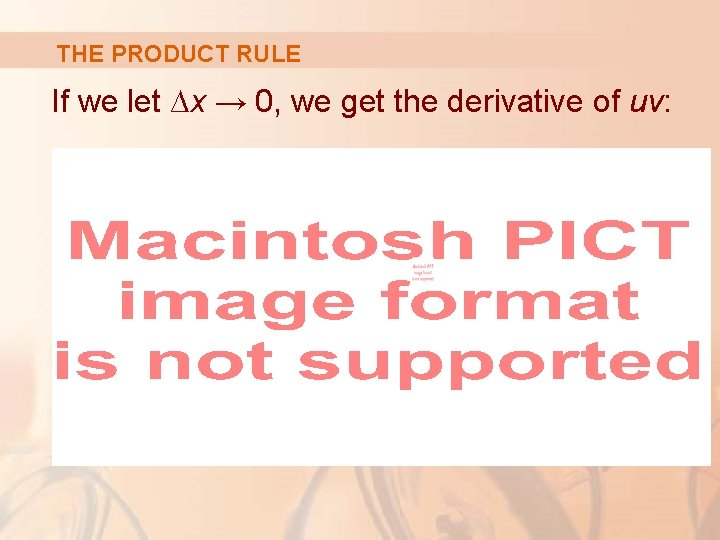THE PRODUCT RULE If we let ∆x → 0, we get the derivative of uv: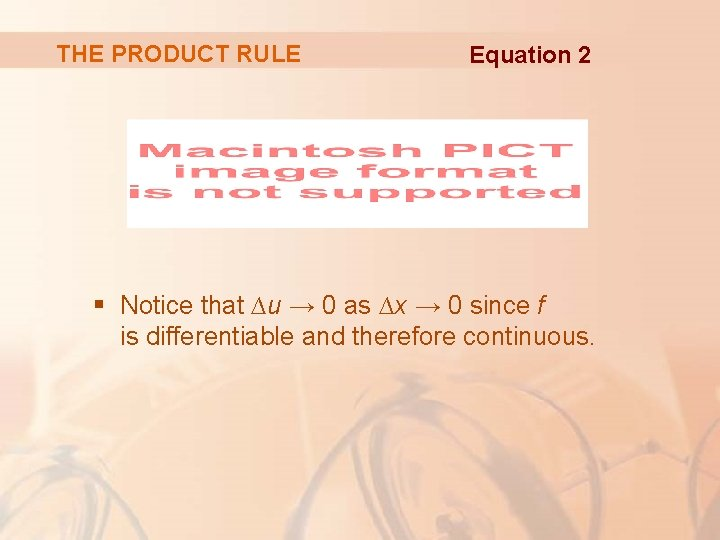THE PRODUCT RULE Equation 2 § Notice that ∆u → 0 as ∆x → 0 since f is differentiable and therefore continuous.THE PRODUCT RULE Though we began by assuming (for the geometric interpretation) that all quantities are positive, we see Equation 1 is always true. § The algebra is valid whether u, v, ∆u, and ∆v are positive or negative. § So, we have proved Equation 2—known as the Product Rule—for all differentiable functions u and v.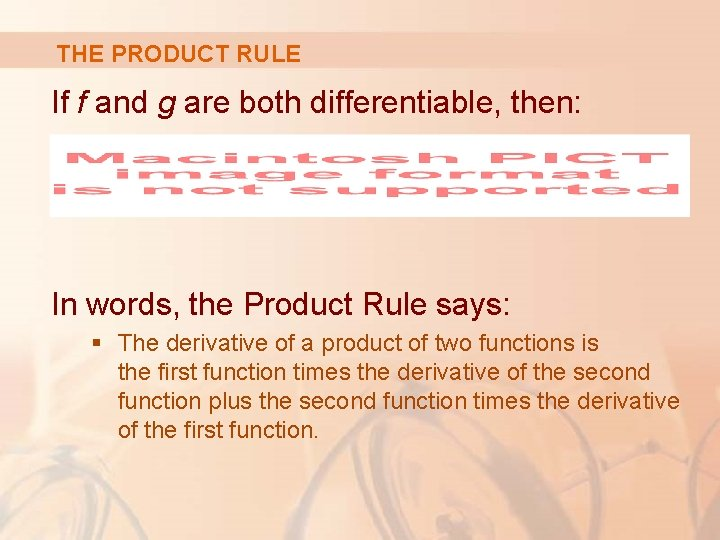THE PRODUCT RULE If f and g are both differentiable, then: In words, the Product Rule says: § The derivative of a product of two functions is the first function times the derivative of the second function plus the second function times the derivative of the first function.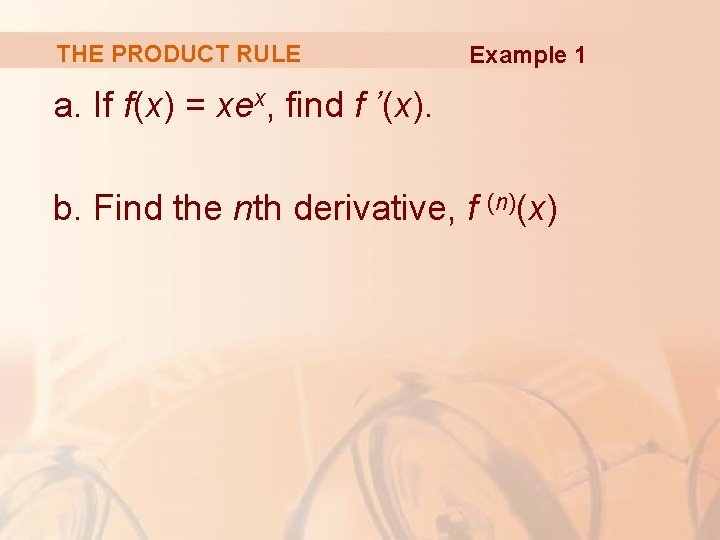THE PRODUCT RULE Example 1 a. If f(x) = xex, find f ’(x). b. Find the nth derivative, f (n)(x)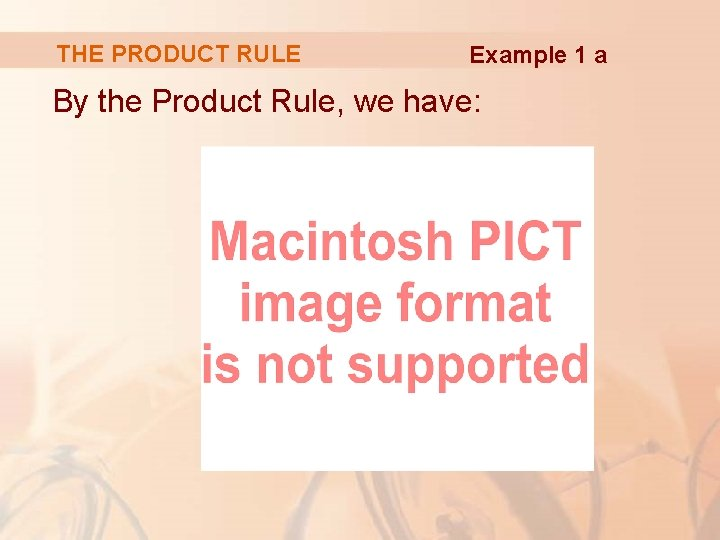THE PRODUCT RULE Example 1 a By the Product Rule, we have: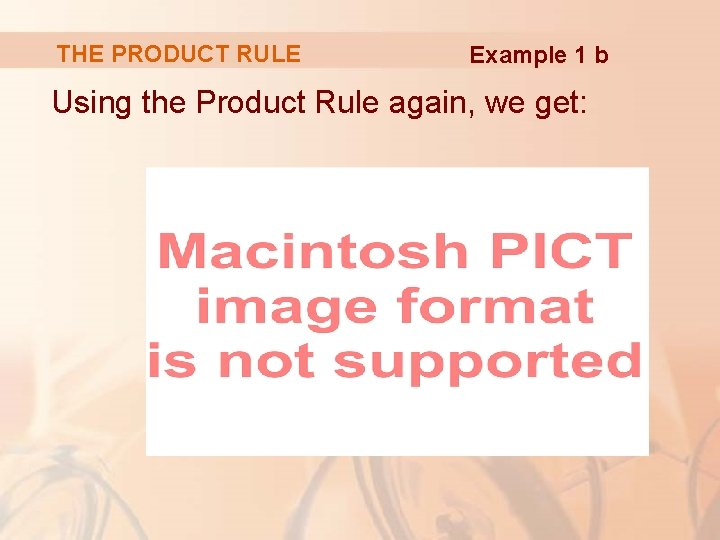THE PRODUCT RULE Example 1 b Using the Product Rule again, we get:THE PRODUCT RULE Example 1 b Further applications of the Product Rule give:THE PRODUCT RULE Example 1 b In fact, each successive differentiation adds another term ex. So:THE PRODUCT RULE Example 2 Differentiate the functionTHE PRODUCT RULE E. g. 2—Solution 1 Using the Product Rule, we have: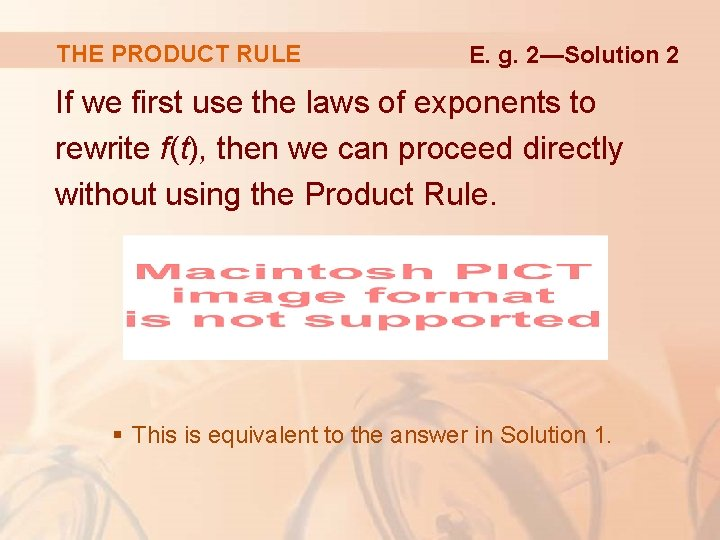THE PRODUCT RULE E. g. 2—Solution 2 If we first use the laws of exponents to rewrite f(t), then we can proceed directly without using the Product Rule. § This is equivalent to the answer in Solution 1.THE PRODUCT RULE Example 2 shows that it is sometimes easier to simplify a product of functions than to use the Product Rule. In Example 1, however, the Product Rule is the only possible method.THE PRODUCT RULE Example 3 If , where g(4) = 2 and g’(4) = 3, find f’(4).THE PRODUCT RULE Example 3 Applying the Product Rule, we get: So,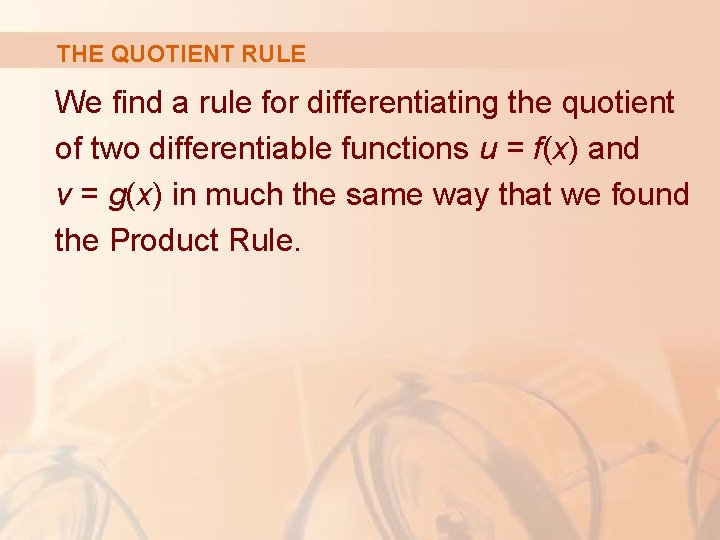THE QUOTIENT RULE We find a rule for differentiating the quotient of two differentiable functions u = f(x) and v = g(x) in much the same way that we found the Product Rule.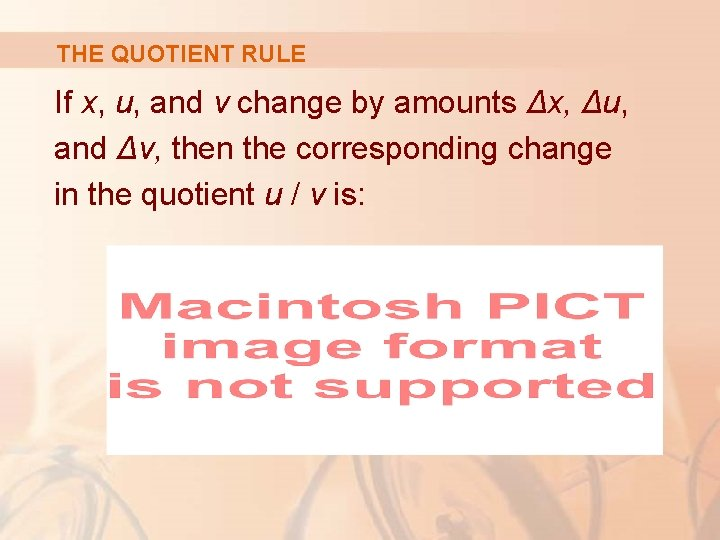THE QUOTIENT RULE If x, u, and v change by amounts Δx, Δu, and Δv, then the corresponding change in the quotient u / v is: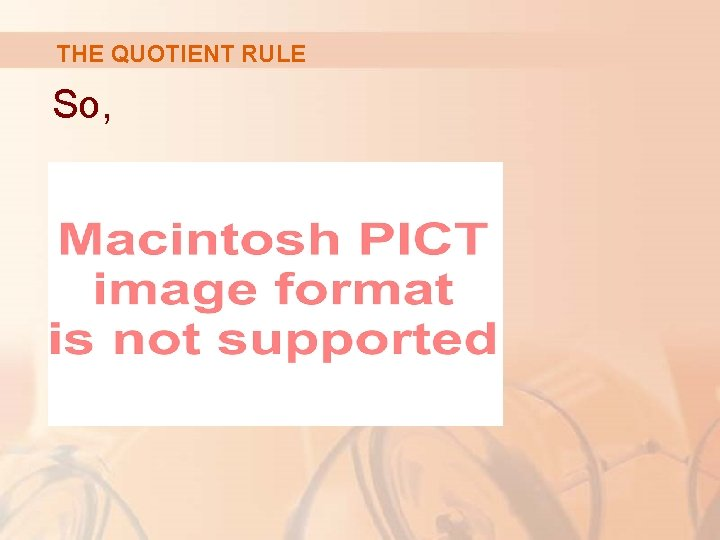THE QUOTIENT RULE So,THE QUOTIENT RULE As ∆x → 0, ∆v → 0 also—because v = g(x) is differentiable and therefore continuous. Thus, using the Limit Laws, we get: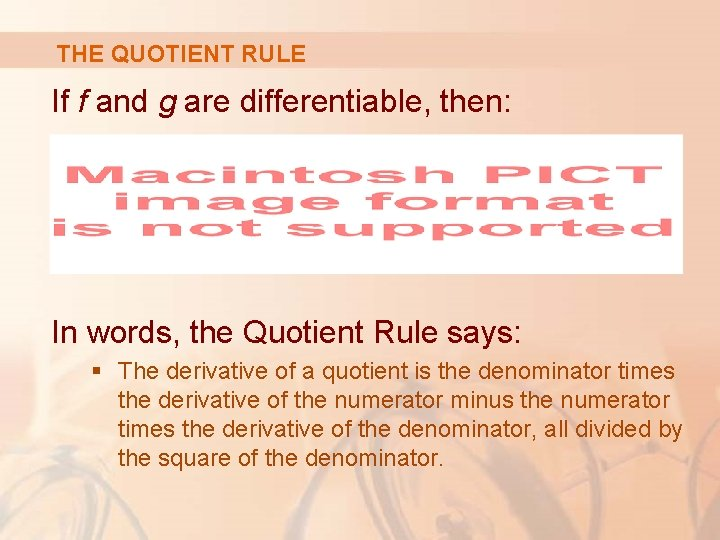THE QUOTIENT RULE If f and g are differentiable, then: In words, the Quotient Rule says: § The derivative of a quotient is the denominator times the derivative of the numerator minus the numerator times the derivative of the denominator, all divided by the square of the denominator.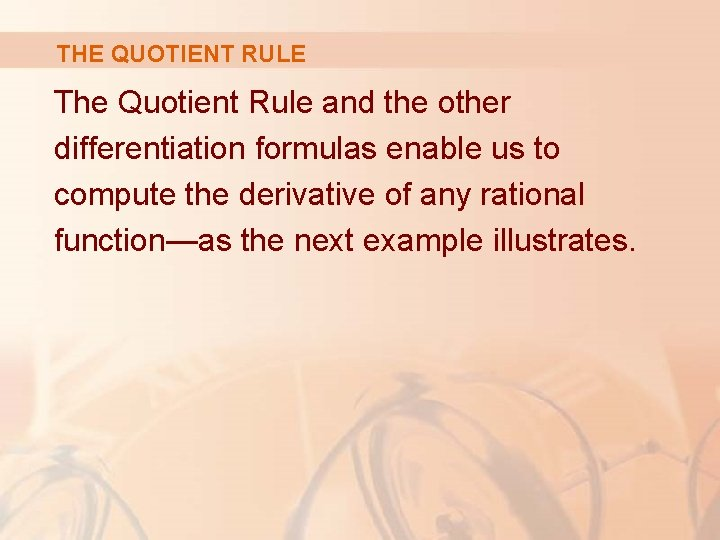THE QUOTIENT RULE The Quotient Rule and the other differentiation formulas enable us to compute the derivative of any rational function—as the next example illustrates.THE QUOTIENT RULE Let Example 4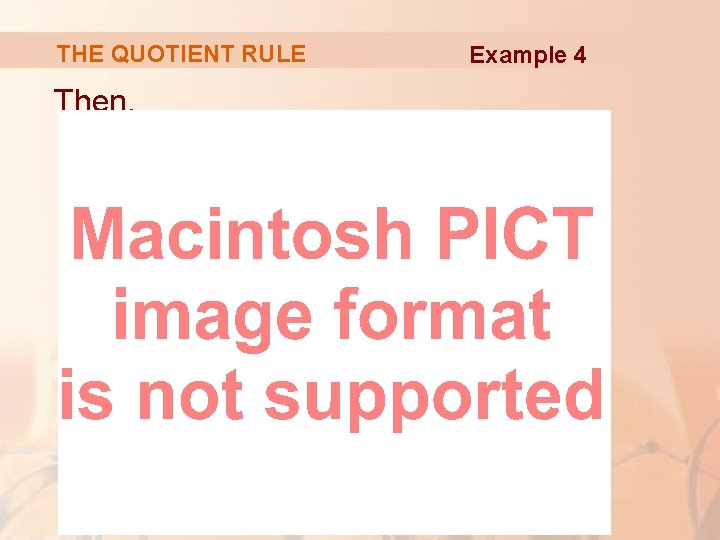THE QUOTIENT RULE Then, Example 4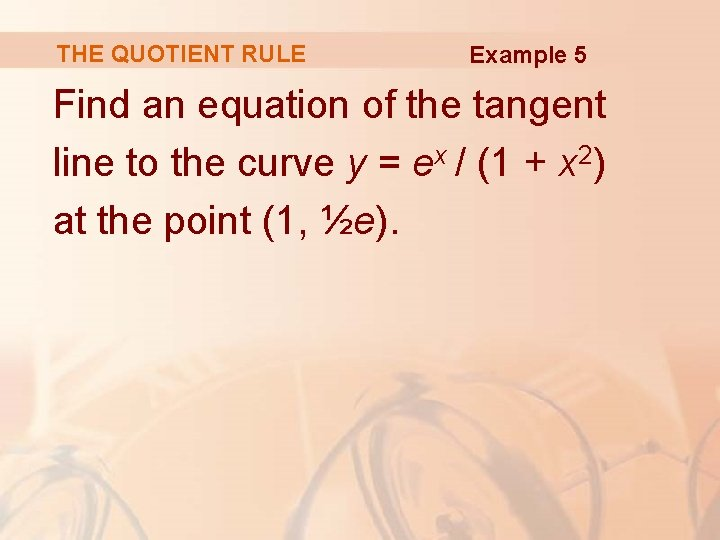THE QUOTIENT RULE Example 5 Find an equation of the tangent line to the curve y = ex / (1 + x 2) at the point (1, ½e).THE QUOTIENT RULE Example 5 According to the Quotient Rule, we have:THE QUOTIENT RULE Example 5 So, the slope of the tangent line at (1, ½e) is: § This means that the tangent line at (1, ½e) is horizontal and its equation is y = ½e.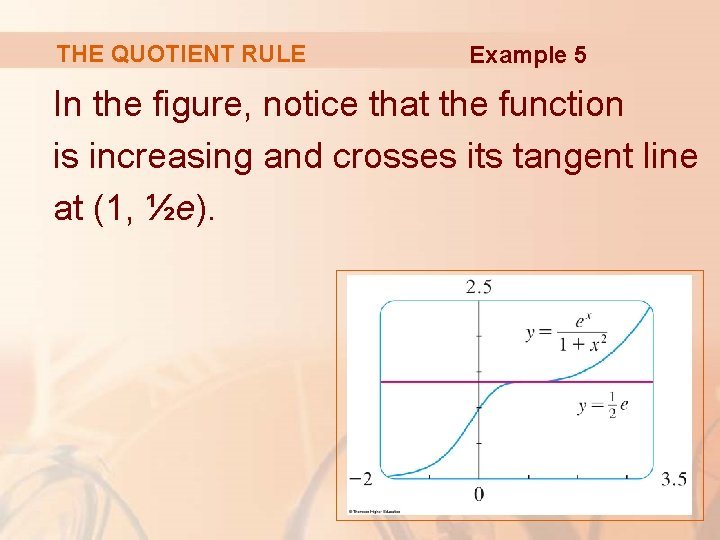THE QUOTIENT RULE Example 5 In the figure, notice that the function is increasing and crosses its tangent line at (1, ½e).NOTE Don’t use the Quotient Rule every time you see a quotient. § Sometimes, it’s easier to rewrite a quotient first to put it in a form that is simpler for the purpose of differentiation.NOTE For instance, though it is possible to differentiate the function using the Quotient Rule, it is much easier to perform the division first and write the function as before differentiating.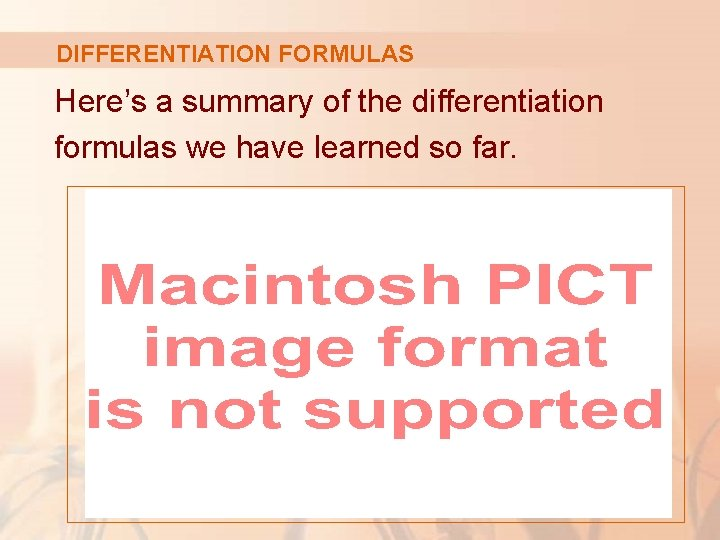DIFFERENTIATION FORMULAS Here’s a summary of the differentiation formulas we have learned so far.